Your data analysis solution

# Weibull model in Excel tutorial

2017-10-20

This tutorial will show you how to set up and interpret a Weibull model – Parametric Survival Regression - in Excel using the XLSTAT software.

## Dataset to run a Weibull model, or parametric survival regression

An Excel sheet with both the data and results can be downloaded by clicking here.

The data have been obtained in Kalbfleisch and Prentice (The Statistical Analysis of Failure Time Data, Wiley, 2002, p. 119) and represent a clinical trial investigating the effect of covariates on time to death of patients with lung cancer. Our goal is to determine which covariate influences the survival time.

## Parametric survival model (Weibull model)

The parametric survival model is based on a classical regression scheme with an underlying distribuion function. The estimation of the model is performed with a maximum likelihood estimation.

In the dataset, the daysurv variable is the time data; the censoring variable is the status variable (1 for death, 0 for censored). The covariates are the performance status of the patient at the beginning of the study (perfstatus), the age of the patient at the beginning of the study (age), the number of month since lung cancer diagnostic at the beginning of the study (month) and the presence of an earlier treatment.

We suppose that the survival function follows a Weibull distribution and want to fit that model.

## Setting up a Weibull model

After opening XLSTAT, select the XLSTAT / Survival analysis / Parametric survival regression command.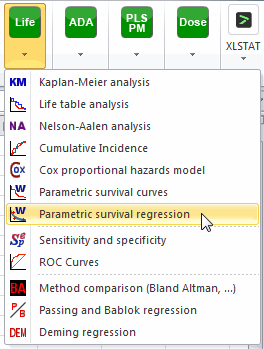Once you've clicked on the button, the Parametric survival regression box will appear. Select the data on the Excel sheet. The Time data corresponds to the durations when the patients either died or were censored. The "Status indicator" describes whether a patient died (event code=1) or was censored (censored code = 0) at a given time.

The covariates are all quantitative and can be selected in the quantitative box. The distribution chosen is the Weibull distribution.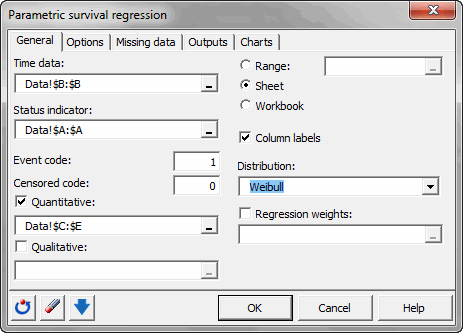Other options can be selected on the other tabs of the dialog box like individual residuals computation, model selection...

The computations begin once you have clicked on OK. The results will then be displayed on a new Excel sheet.

## Interpreting the results of a parametric survival model

The first table displays a summary of the data. We can see that the number of observed times (time steps) is different than the number of observations.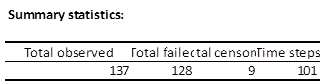The next table gives several indicators of the quality of the model (or goodness of fit). These results are equivalent to the R2 and to the analysis of variance table in linear regression. The most important value to look at is the probability of Chi-square test on the log ratio. This is equivalent to the Fisher's F test: we try to evaluate if the variables bring significant information by comparing the model as it is defined with a simpler model with no impact of the covariates. In this case, as the probability is lower than 0.0001, we can conclude that significant information is brought by the variables.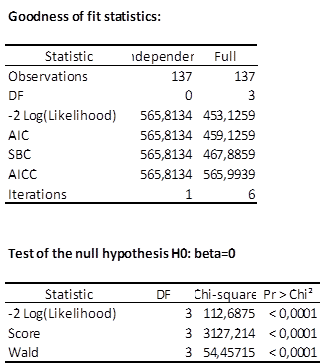The following table gives details on the model. This table is helpful in understanding the effect of the various variables and parameters of the Weibull distribution.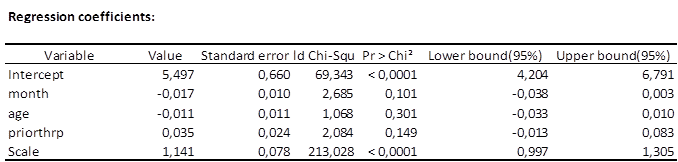On this table we can see that the intercept and scale parameters have a significant effect. The model fits well to a Weibull distribution. The explanatory variables do not have a significant effect on the model.

Finally, the cumulative survival function is displayed with both empirical values and theoretical values. We can see that the Weibull distribution seems to be a good choice to fit this regression model.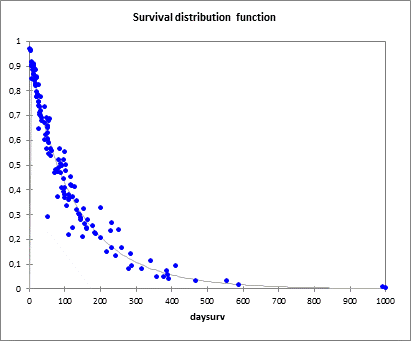This study has shown that the Weibull distribution seems to be a good choice and the estimated values fit well the theoretical values (when all covariates are at their mean value).

### Contact Us

#### Contact our technical support team: support@xlstat.com

https://cdn.desk.com/
false
desk
Loading
seconds ago
a minute ago
minutes ago
an hour ago
hours ago
a day ago
days ago
about
false
Invalid characters found
/customer/portal/articles/autocomplete
9283